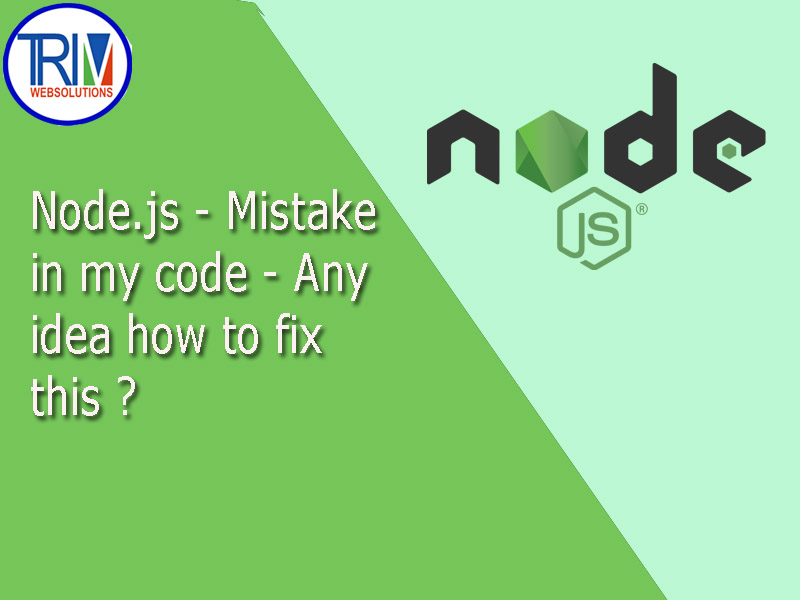Quick Inquiry

# Mistake in my code - Any idea how to fix this in Node.js ?As far as I can tell, there are two significant issues:

1. `range = range.push(i);` There is no value returned by the push method. As a result, you should not assign a value to the range variable in this case.
2. `l.reduce(multiply);` The variable 'l' is not defined in this case. This variable must be replaced with the 'range' variable.

Please find the following code, which has been updated and is now working:

``` ```'use strict';

module.exports.multiplyRange = (event, context, callback) => {

var start = event.start;
var end = event.end;

// This is where I'm having problems

var range = [];
for (var i = start; i <= end; i++) {
range.push(i);
}

let multiply = (a, b) => a + b;
var result = range.reduce(multiply);

const response = {
statusCode: 200,
'Access-Control-Allow-Origin': '*', // Required for CORS support to work
},
body: {
result: result,
},
};
callback(null, response);
};``````